# Difference between revisions of "Substantive derivative"

The substantive derivative is a derivative taken along a path moving with velocity v, and is often used in fluid mechanics and classical mechanics. It describes the time rate of change of some quantity (such as heat or momentum) by following it, while moving with a – space and time dependent – velocity field.

The material derivative of a scalar field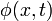$\phi( x, t )$ is: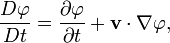$\frac{D\varphi}{Dt} = \frac{\partial \varphi}{\partial t} + \mathbf{v}\cdot\nabla \varphi,$

where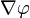$\nabla \varphi$ is the gradient of the scalar.

For a vector field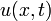$u( x, t )$ it is defined as: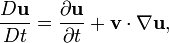$\frac{D\mathbf{u}}{Dt} = \frac{\partial \mathbf{u}}{\partial t} + \mathbf{v}\cdot\nabla \mathbf{u},$

where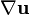$\nabla \mathbf{u}$ is the covariant derivative of a vector.

In case of the material derivative of a vector field, the term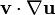$\mathbf{v} \cdot \nabla \mathbf{u}$ can both be interpreted as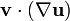$\mathbf{v} \cdot (\nabla \mathbf{u})$, involving the tensor derivative of u, or as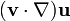$(\mathbf{v} \cdot \nabla) \mathbf{u}$, leading to the same result.

## Names

There are many other names for this operator, including:

• material derivative
• convective derivative